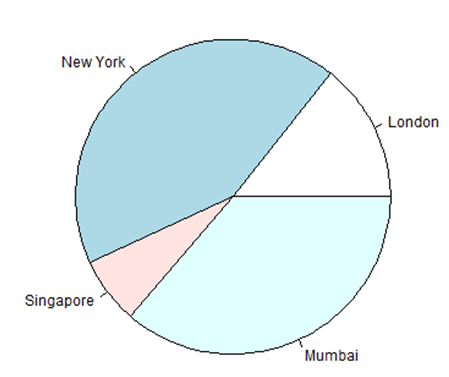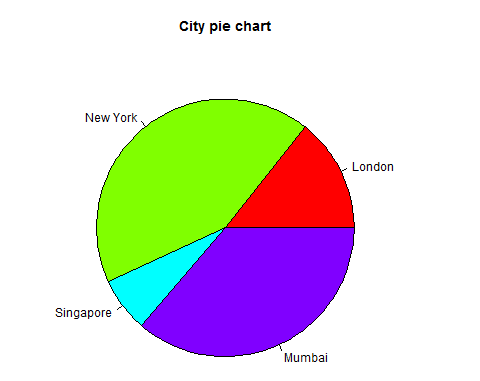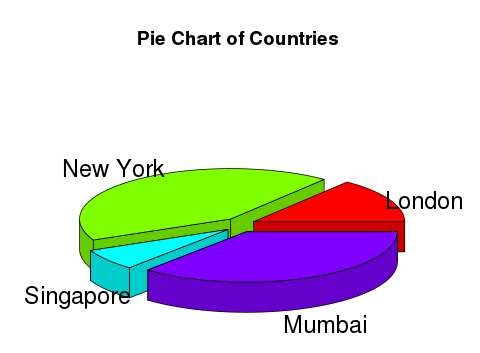# R语言 饼状图

R编程语言有许多库来创建图表和图表。 饼图是将值表示为具有不同颜色的圆的切片。 切片被标记，并且对应于每个片的数字也在图表中表示。

## 语法

```pie(x, labels, radius, main, col, clockwise)
```

• x是包含饼图中使用的数值的向量。
• labels用于给出切片的描述。
• main表示图表的标题。
• col表示调色板。
• clockwise是指示片段是顺时针还是逆时针绘制的逻辑值。

## 例

```# Create data for the graph.
x <- c(21, 62, 10, 53)
labels <- c("London", "New York", "Singapore", "Mumbai")

# Give the chart file a name.
png(file = "city.jpg")

# Plot the chart.
pie(x,labels)

# Save the file.
dev.off()
```## 例

```# Create data for the graph.
x <- c(21, 62, 10, 53)
labels <- c("London", "New York", "Singapore", "Mumbai")

# Give the chart file a name.
png(file = "city_title_colours.jpg")

# Plot the chart with title and rainbow color pallet.
pie(x, labels, main = "City pie chart", col = rainbow(length(x)))

# Save the file.
dev.off()
```## 切片百分比和图表图例

```# Create data for the graph.
x <-  c(21, 62, 10,53)
labels <-  c("London","New York","Singapore","Mumbai")

piepercent<- round(100*x/sum(x), 1)

# Give the chart file a name.
png(file = "city_percentage_legends.jpg")

# Plot the chart.
pie(x, labels = piepercent, main = "City pie chart",col = rainbow(length(x)))
legend("topright", c("London","New York","Singapore","Mumbai"), cex = 0.8,
fill = rainbow(length(x)))

# Save the file.
dev.off()
```## 3D饼图

```# Get the library.
library(plotrix)

# Create data for the graph.
x <-  c(21, 62, 10,53)
lbl <-  c("London","New York","Singapore","Mumbai")

# Give the chart file a name.
png(file = "3d_pie_chart.jpg")

# Plot the chart.
pie3D(x,labels = lbl,explode = 0.1, main = "Pie Chart of Countries ")

# Save the file.
dev.off()
```## 【阿里云】棒款特惠精选

#### 云服务器低至8.1元/月 - 开始搭建你的博客吧